Anzeige

# Causality in special relativity

24. Jan 2015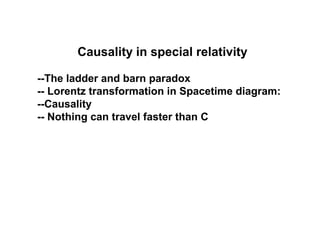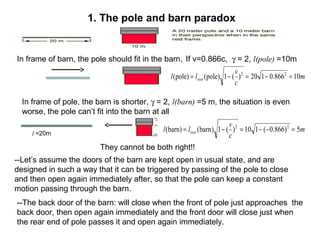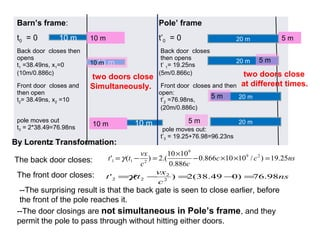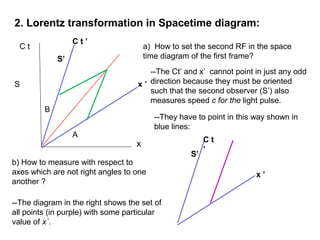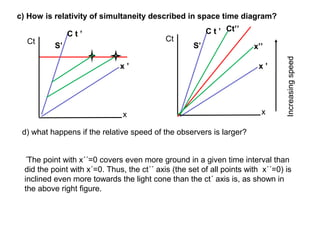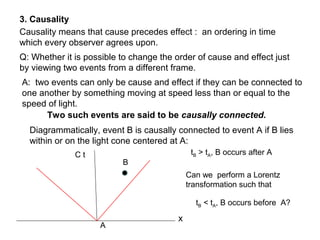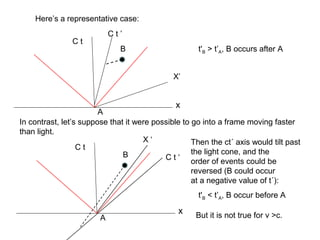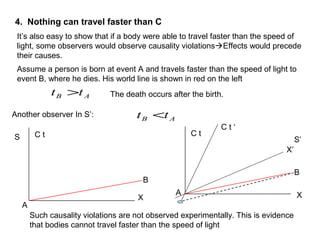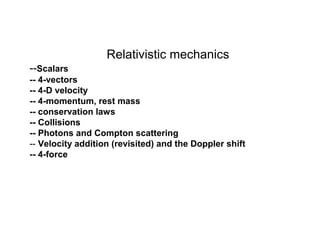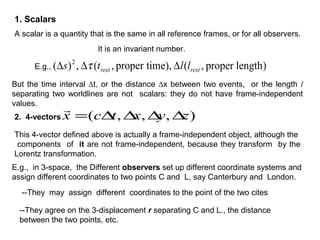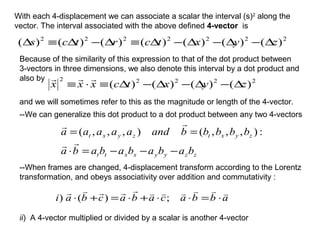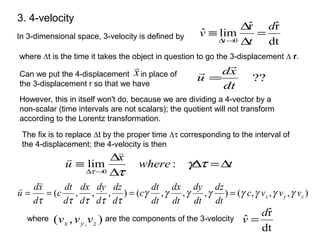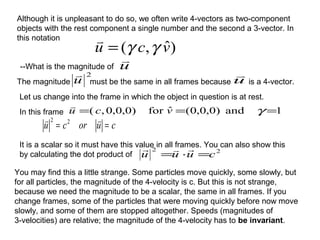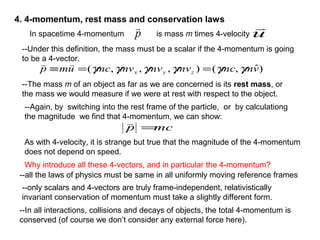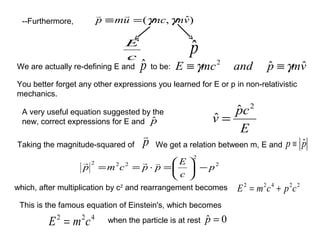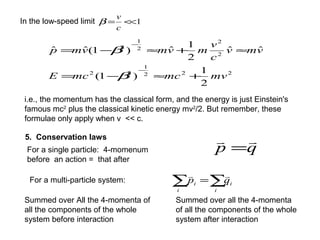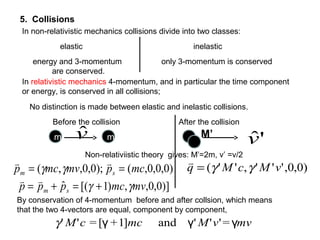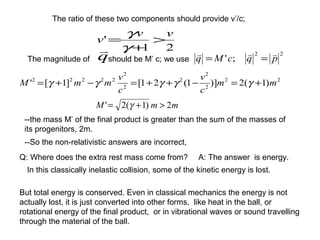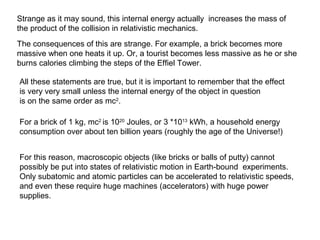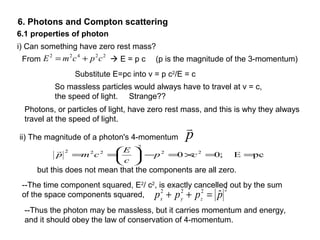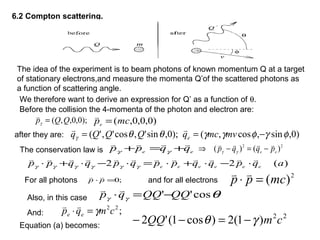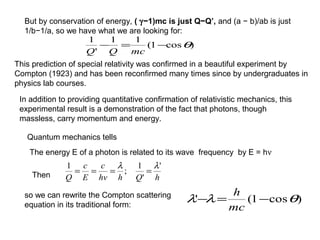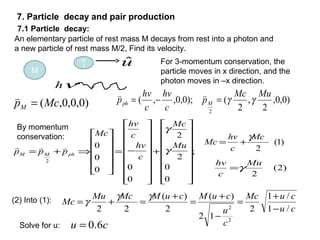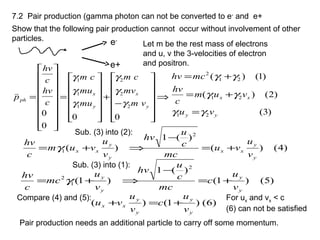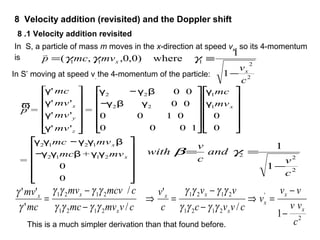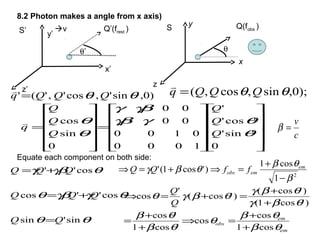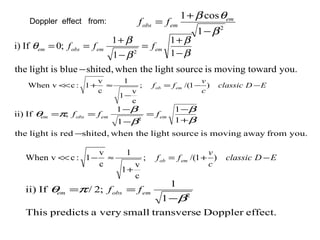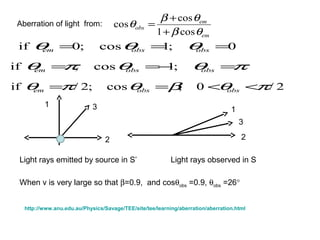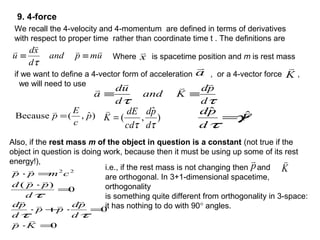1 von 29
Anzeige

### Causality in special relativity

1. Causality in special relativity --The ladder and barn paradox -- Lorentz transformation in Spacetime diagram: --Causality -- Nothing can travel faster than C
2. 1. The pole and barn paradox If v=0.866c, γ = 2, l(pole) =10mIn frame of barn, the pole should fit in the barn, m c v ll rest 10866.0120)(1)pole()pole( 22 =−=−= l =20m In frame of pole, the barn is shorter, γ = 2, l(barn) =5 m, the situation is even worse, the pole can’t fit into the barn at all m c v ll rest 5)866.0(110)(1)barn((barn) 22 =−−=−= --The back door of the barn: will close when the front of pole just approaches the back door, then open again immediately and the front door will close just when the rear end of pole passes it and open again immediately. They cannot be both right!! --Let’s assume the doors of the barn are kept open in usual state, and are designed in such a way that it can be triggered by passing of the pole to close and then open again immediately after, so that the pole can keep a constant motion passing through the barn.
3. Barn’s frame: Pole’ frame t0 = 0 t’0 = 0 Back door closes then opens t1 =38.49ns, x1=0 (10m/0.886c) Back door closes then opens t’ 1= 19.25ns (5m/0.866c) Front door closes and then open t2= 38.49ns, x2 =10 Front door closes and then open: t’2 =76.98ns, (20m/0.886c) pole moves out t3 = 2*38.49=76.98ns pole moves out: t’3 = 19.25+76.98=96.23ns 10 m 10 m10 m 10 m 10 m 10 m 5 m20 m 20 m 20 m 5 m 5 m 20 m5 m The front door closes: The back door closes: --The door closings are not simultaneous in Pole’s frame, and they permit the pole to pass through without hitting either doors. --The surprising result is that the back gate is seen to close earlier, before the front of the pole reaches it. By Lorentz Transformation: nscc cc vx tt 25.19)/1010866.0 886.0 1010 .(2)(' 29 9 211 =××− × =−= γ ns c vx tt 98.76)049.38(2)(' 2 2 22 =−=−=γ two doors close Simultaneously. two doors close at different times.
4. 2. Lorentz transformation in Spacetime diagram: C t x S C t ’ x ’ S’ --The Ct’ and x’ cannot point in just any odd direction because they must be oriented such that the second observer (S’) also measures speed c for the light pulse. a) How to set the second RF in the space time diagram of the first frame? --They have to point in this way shown in blue lines: A B b) How to measure with respect to axes which are not right angles to one another ? --The diagram in the right shows the set of all points (in purple) with some particular value of x´. C t ’ x ’ S’
5. c) How is relativity of simultaneity described in space time diagram? C t ’ x ’ S’ Ct x d) what happens if the relative speed of the observers is larger? C t ’ x ’ S’ Ct x Ct’’ x’’ ´The point with x´´=0 covers even more ground in a given time interval than did the point with x´=0. Thus, the ct´´ axis (the set of all points with x´´=0) is inclined even more towards the light cone than the ct´ axis is, as shown in the above right figure. Increasingspeed
6. 3. Causality Causality means that cause precedes effect : an ordering in time which every observer agrees upon. Q: Whether it is possible to change the order of cause and effect just by viewing two events from a different frame. A: two events can only be cause and effect if they can be connected to one another by something moving at speed less than or equal to the speed of light. Two such events are said to be causally connected. Diagrammatically, event B is causally connected to event A if B lies within or on the light cone centered at A: A B C t x tB > tA, B occurs after A Can we perform a Lorentz transformation such that tB < tA, B occurs before A?
7. Here’s a representative case: A B C t x A B C t x X’ C t ’ In contrast, let’s suppose that it were possible to go into a frame moving faster than light. Then the ct´ axis would tilt past the light cone, and the order of events could be reversed (B could occur at a negative value of t´): C t ‘ X ‘ t'B > t’A, B occurs after A But it is not true for v >c. t'B < t’A, B occur before A
8. It’s also easy to show that if a body were able to travel faster than the speed of light, some observers would observe causality violationsEffects would precede their causes. A X B C t Such causality violations are not observed experimentally. This is evidence that bodies cannot travel faster than the speed of light AB tt > S Assume a person is born at event A and travels faster than the speed of light to event B, where he dies. His world line is shown in red on the left B X C t C t ‘ X’ A S’ Another observer In S’: AB tt < 4. Nothing can travel faster than C The death occurs after the birth.
9. Relativistic mechanics --Scalars -- 4-vectors -- 4-D velocity -- 4-momentum, rest mass -- conservation laws -- Collisions -- Photons and Compton scattering -- Velocity addition (revisited) and the Doppler shift -- 4-force
10. 1. Scalars A scalar is a quantity that is the same in all reference frames, or for all observers. It is an invariant number. E.g., )lengthproper,(),timeproper,(,)( 2 restrest llts ∆∆∆ τ But the time interval ∆t, or the distance ∆x between two events, or the length l separating two worldlines are not scalars: they do not have frame-independent values. 2. 4-vectors ),,,( zyxtcx ∆∆∆∆=  This 4-vector defined above is actually a frame-independent object, although the components of it are not frame-independent, because they transform by the Lorentz transformation. E.g., in 3-space, the Different observers set up different coordinate systems and assign different coordinates to two points C and L, say Canterbury and London. --They may assign different coordinates to the point of the two cites --They agree on the 3-displacement r separating C and L., the distance between the two points, etc.
11. With each 4-displacement we can associate a scalar the interval (s)2 along the vector. The interval associated with the above defined 4-vector is 2222222 )()()()()()()( zyxtcrtcs ∆−∆−∆−∆≡∆−∆≡∆ Because of the similarity of this expression to that of the dot product between 3-vectors in three dimensions, we also denote this interval by a dot product and also by 22222 )()()()( zyxtcxxx ∆−∆−∆−∆≡⋅≡  and we will sometimes refer to this as the magnitude or length of the 4-vector. --We can generalize this dot product to a dot product between any two 4-vectors zzyyxxtt zyxtzyxt bababababa bbbbbandaaaaa −−−=⋅ ==   :),,,(),,,( --When frames are changed, 4-displacement transform according to the Lorentz transformation, and obeys associativity over addition and commutativity : abbacabacbai  ⋅=⋅⋅+⋅=+⋅ ;)() ii) A 4-vector multiplied or divided by a scalar is another 4-vector
12. 3. 4-velocity In 3-dimensional space, 3-velocity is defined by dt rˆrˆ limˆ 0 d t v t = ∆ ∆ ≡ →∆ where ∆t is the time it takes the object in question to go the 3-displacement ∆ r. However, this in itself won't do, because we are dividing a 4-vector by a non-scalar (time intervals are not scalars); the quotient will not transform according to the Lorentz transformation. ?? dt xd u   =Can we put the 4-displacement in place of the 3-displacement r so that we have The fix is to replace ∆t by the proper time ∆τ corresponding to the interval of the 4-displacement; the 4-velocity is then twhere x u ∆=∆ ∆ ∆ ≡ →∆ τγ ττ :lim 0   ),,,(),,,(),,,( zyx vvvc dt dz dt dy dt dx dt dt c d dz d dy d dx d dt c d xd u γγγγγγγγ τττττ ====   ),( , zyx vvvwhere are the components of the 3-velocity dt rˆ ˆ d v = x 
13. Although it is unpleasant to do so, we often write 4-vectors as two-component objects with the rest component a single number and the second a 3-vector. In this notation )ˆ,( vcu γγ=  --What is the magnitude of u  The magnitude must be the same in all frames because 2 u  is a 4-vector.u  Let us change into the frame in which the object in question is at rest. In this frame 1and)0,0,0(ˆfor)0,0,0,( === γvcu  cuorcu ==  22 It is a scalar so it must have this value in all frames. You can also show this by calculating the dot product of You may find this a little strange. Some particles move quickly, some slowly, but for all particles, the magnitude of the 4-velocity is c. But this is not strange, because we need the magnitude to be a scalar, the same in all frames. If you change frames, some of the particles that were moving quickly before now move slowly, and some of them are stopped altogether. Speeds (magnitudes of 3-velocities) are relative; the magnitude of the 4-velocity has to be invariant. 22 cuuu =⋅= 
14. 4. 4-momentum, rest mass and conservation laws In spacetime 4-momentum is mass m times 4-velocityp  u  --Under this definition, the mass must be a scalar if the 4-momentum is going to be a 4-vector. --The mass m of an object as far as we are concerned is its rest mass, or the mass we would measure if we were at rest with respect to the object. )ˆ,(),,,( vmmcmvmvmvmcump zyx γγγγγγ ==≡  --Again, by switching into the rest frame of the particle, or by calculationg the magnitude we find that 4-momentum, we can show: mcp =  As with 4-velocity, it is strange but true that the magnitude of the 4-momentum does not depend on speed. Why introduce all these 4-vectors, and in particular the 4-momentum? --all the laws of physics must be same in all uniformly moving reference frames --only scalars and 4-vectors are truly frame-independent, relativistically invariant conservation of momentum must take a slightly different form. --In all interactions, collisions and decays of objects, the total 4-momentum is conserved (of course we don’t consider any external force here).
15. --Furthermore, )ˆ,( vmmcump γγ=≡  c E pˆ We are actually re-defining E and pˆ to be: vmpandmcE ˆˆ2 γγ ≡≡ You better forget any other expressions you learned for E or p in non-relativistic mechanics. A very useful equation suggested by the new, correct expressions for E and pˆ E cp v 2 ˆ ˆ = Taking the magnitude-squared of p  We get a relation between m, E and pp ˆ≡ 2 2 222 p c E ppcmp −      =⋅==  which, after multiplication by c2 and rearrangement becomes 22422 cpcmE += This is the famous equation of Einstein's, which becomes 422 cmE = when the particle is at rest 0ˆ =p
16. In the low-speed limit 1<<= c v β 222 1 22 2 2 2 1 2 2 1 )1( ˆˆ 2 1 ˆ)1(ˆˆ mvmcmcE vmv c v mvmvmp +≈−= ≈+≈−= − − β β i.e., the momentum has the classical form, and the energy is just Einstein's famous mc2 plus the classical kinetic energy mv2 /2. But remember, these formulae only apply when v << c. 5. Conservation laws qp  = Summed over All the 4-momenta of all the components of the whole system before interaction Summed over all the 4-momenta of all the components of the whole system after interaction ∑∑ = i i i i qp  For a single particle: 4-momenum before an action = that after For a multi-particle system:
17. 5. Collisions In non-relativistic mechanics collisions divide into two classes: elastic inelastic energy and 3-momentum are conserved. only 3-momentum is conserved In relativistic mechanics 4-momentum, and in particular the time component or energy, is conserved in all collisions; No distinction is made between elastic and inelastic collisions. m m Before the collision After the collision )0,0,0,();0,0,,( mcpmvmcp sm ==  γγ vˆ M’ 'ˆv )]0,0,,)1[(ˆ mvmcppp sm γγ +=+=  )0,0,''',''( vMcMq γγ=  By conservation of 4-momentum before and after collsion, which means that the two 4-vectors are equal, component by component, γ' M'c = [γ +1]mc and γ' M'v'= γmv Non-relativiistic theory gives: M’=2m, v’ =v/2
18. The ratio of these two components should provide v’/c; 21 ' vv v > + = γ γ The magnitude of q  should be M’ c; we use 22 2 2 2 2 2 22222 )1(2)]1(21[]1[' mm c v c v mmM +=−++=−+= γγγγγ mmM 2)1(2' >+= γ --So the non-relativistic answers are incorrect, --the mass M’ of the final product is greater than the sum of the masses of its progenitors, 2m. Q: Where does the extra rest mass come from? A: The answer is energy. In this classically inelastic collision, some of the kinetic energy is lost. But total energy is conserved. Even in classical mechanics the energy is not actually lost, it is just converted into other forms, like heat in the ball, or rotational energy of the final product, or in vibrational waves or sound travelling through the material of the ball. 22 ;' pqcMq  ==
19. Strange as it may sound, this internal energy actually increases the mass of the product of the collision in relativistic mechanics. The consequences of this are strange. For example, a brick becomes more massive when one heats it up. Or, a tourist becomes less massive as he or she burns calories climbing the steps of the Effiel Tower. All these statements are true, but it is important to remember that the effect is very very small unless the internal energy of the object in question is on the same order as mc2 . For a brick of 1 kg, mc2 is 1020 Joules, or 3 *1013 kWh, a household energy consumption over about ten billion years (roughly the age of the Universe!) For this reason, macroscopic objects (like bricks or balls of putty) cannot possibly be put into states of relativistic motion in Earth-bound experiments. Only subatomic and atomic particles can be accelerated to relativistic speeds, and even these require huge machines (accelerators) with huge power supplies.
20. 6. Photons and Compton scattering i) Can something have zero rest mass? Substitute E=pc into v = p c2 /E = c 6.1 properties of photon 22422 cpcmE +=From  E = p c (p is the magnitude of the 3-momentum) So massless particles would always have to travel at v = c, the speed of light. Strange?? Photons, or particles of light, have zero rest mass, and this is why they always travel at the speed of light. ii) The magnitude of a photon's 4-momentum p  pcE;00 22 2 222 ==×=−      == cp c E cmp  but this does not mean that the components are all zero. --The time component squared, E2 / c2 , is exactly cancelled out by the sum of the space components squared, 2222 ˆpppp zyx =++ --Thus the photon may be massless, but it carries momentum and energy, and it should obey the law of conservation of 4-momentum.
21. 6.2 Compton scattering. The idea of the experiment is to beam photons of known momentum Q at a target of stationary electrons,and measure the momenta Q’of the scattered photons as a function of scattering angle. We therefore want to derive an expression for Q’ as a function of θ. Before the collision the 4-momenta of the photon and electron are: );0,0,,( QQp =γ  )0,0,0,(mcpe =  after they are: )0,sin,cos,();0,sin',cos','( φγφγγθθγ −== mvmcqQQQq e  The conservation law is ee qqpp  +=+ γγ 22 )()( ee pqqp  −=−⇒ γγ )(22 aqpqqppqpqqpp eeeeee  ⋅−⋅+⋅=⋅−⋅+⋅ γγγγγγ For all photons ;0=⋅ pp  and for all electrons 2 )(mcpp =⋅  Also, in this case θγγ cos'' QQQQqp −=⋅  ;22 cmqp ee γ=⋅  And: 22 )1(2)cos1('2 cmQQ γθ −=−−Equation (a) becomes:
22. But by conservation of energy, ( γ−1)mc is just Q−Q’, and (a − b)/ab is just 1/b−1/a, so we have what we are looking for: )cos1( 11 ' 1 θ−=− mcQQ hQhhv c E c Q ' ' 1 ; 1 λλ ==== This prediction of special relativity was confirmed in a beautiful experiment by Compton (1923) and has been reconfirmed many times since by undergraduates in physics lab courses. In addition to providing quantitative confirmation of relativistic mechanics, this experimental result is a demonstration of the fact that photons, though massless, carry momentum and energy. Quantum mechanics tells The energy E of a photon is related to its wave frequency by E = hν so we can rewrite the Compton scattering equation in its traditional form: Then )cos1(' θλλ −=− mc h
23. An elementary particle of rest mass M decays from rest into a photon and a new particle of rest mass M/2, Find its velocity. M M/ 2 uˆ νh For 3-momentum conservation, the particle moves in x direction, and the photon moves in –x direction. )0,0,0,(McpM =  )0,0, 2 , 2 ();0,0,,( 2 MuMc p c hv c hv p Mph γγ=−=  ; 0 0 2 2 0 00 0 0 2                   +                   −=             ⇒+= Mu Mc c hv c hv Mc ppp phMM γ γ  )2( 2 Mu c hv γ= )1( 2 Mc c hv Mc γ += (2) Into (1): cu cuMc c u cuMcuMMcMu Mc /1 /1 2 12 )( 2 )( 22 2 2 − + = − + = + =+= γγ γ 7. Particle decay and pair production cu 6.0=Solve for u: 7.1 Particle decay: By momentum conservation:
24. 7.2 Pair production (gamma photon can not be converted to e- and e+ Show that the following pair production cannot occur without involvement of other particles. e- e+ Let m be the rest mass of electrons and u, v the 3-velocities of electron and positron. ⇒               − +               =                   = 00 0 0 2 2 2 1 1 1 y x y x ph vm mv cm mu mu cm c hv c hv p γ γ γ γ γ γ  )3( )2()( )1()( 21 21 21 2 yy xx vu vum c hv mchv γγ γγ γγ = += += Sub. (3) into (2): )4()( )(1 )( 2 1 y y xx y y xx v u vu mc c u hv v u vum c hv += − ⇒+= γ Sub. (3) into (1): )5()1( )(1 )1( 2 1 2 y y y y v u c mc c u hv v u mc c hv += − ⇒+= γ Compare (4) and (5): )6()1()( y y y y xx v u c v u vu +=+ For ux and vx < c (6) can not be satisfied Pair production needs an additional particle to carry off some momentum.
25. 8 Velocity addition (revisited) and the Doppler shift In S, a particle of mass m moves in the x-direction at speed vx, so its 4-momentum is 2 2111 1 1 where)0,0,,( c v mvmcp x x − ≡= γγγ  In S’ moving at speed v, the 4-momentum of the particle: ϖ p' = γ' mc γ' mv'x γ' mv'y γ' mv'z ⎡ ⎣ ⎢ ⎢ ⎢ ⎢ ⎤ ⎦ ⎥ ⎥ ⎥ ⎥ = γ2 −γ2β 0 0 −γ2β γ2 0 0 0 0 1 0 0 0 0 1 ⎡ ⎣ ⎢ ⎢ ⎢ ⎢ ⎤ ⎦ ⎥ ⎥ ⎥ ⎥ γ1mc γ1mvx 0 0 ⎡ ⎣ ⎢ ⎢ ⎢ ⎢ ⎤ ⎦ ⎥ ⎥ ⎥ ⎥ = γ2γ1mc −γ2γ1mvx β −γ2γ1mcβ + γ1γ2mvx 0 0 ⎡ ⎣ ⎢ ⎢ ⎢ ⎢ ⎤ ⎦ ⎥ ⎥ ⎥ ⎥ 2 22 1 1 c v and c v with − == γβ 2 ' 2121 2121 2121 2121 1 / ' / / ' '' c vv vv v cvvc vv c v cvmvmc cmcvmv mc mv x x x x xx x xx − − =⇒ − − =⇒ − − = γγγγ γγγγ γγγγ γγγγ γ γ 8 .1 Velocity addition revisited This is a much simpler derivation than that found before.
26. 8.2 Photon makes a angle from x axis) θ Q(fobs )S y x z v Q’(frest )S’ y’ x’ z’ );0,sin,cos,( θθ QQQq =  )0,'sin','cos','(' θθ QQQq =  ; 0 'sin' 'cos' ' 1000 0100 00 00 0 sin cos                         =             = θ θγγβ γβγ θ θ Q Q Q Q Q Q q  θ’ Equate each component on both side: 'sin'sin 'cos''cos 'cos'' θθ θγγβθ θγβγ QQ QQQ QQQ = += += 2 1 cos1 )'cos1(' β θβ θβγ − + =⇒+=⇒ em emobs ffQQ em em obs Q Q θβ θβ θ θβ θβ θβγ θβγ θβγθ cos1 cos cos 'cos1 'cos )'cos1( )'cos( )'cos( ' cos + + =⇒ + + = + + =+=⇒ c v =β
27. 2 1 cos1 β θβ − + = em emobs ffDoppler effect from: you.towardmovingissourcelightthewhen,shitedblueislightthe 1 1 1 1 ;0Ifi) 2 − − + = − + == β β β β θ ememobsem fff you.fromawaymovingissourcelightthewhen,shitedredislightthe 1 1 1 1 ;Ifii) 2 − + − = − − == β β β β πθ ememobsem fff effect.DoppleretransverssmallveryapredictsThis 1 1 ;2/Ifii) 2 β πθ − == emobsem ff EDclassic c v ff emob −−= − ≈+<< )1/(; c v 1 1 c v 1:cvWhen EDclassic c v ff emob −+= + ≈−<< )1/(; c v 1 1 c v 1:cvWhen
28. em em obs θβ θβ θ cos1 cos cos + + =Aberration of light from: 0;1cos;0if === obsobsem θθθ πθθπθ =−== obsobsem ;1cos;if 2/0;cos;2/if πθβθπθ <<== obsobsem 1 2 1 2 3 3 Light rays emitted by source in S’ Light rays observed in S When v is very large so that β=0.9, and cosθobs =0.9, θobs =26° http://www.anu.edu.au/Physics/Savage/TEE/site/tee/learning/aberration/aberration.html
29. a  if we want to define a 4-vector form of acceleration , or a 4-vector force , we will need to use K  ττ d pd Kand d ud a   ≡≡ 9. 4-force umpand d xd u    ≡≡ τ We recall the 4-velocity and 4-momentum are defined in terms of derivatives with respect to proper time rather than coordinate time t . The definitions are Where is spacetime position and m is rest massx  )ˆ,(Because p c E p =  ) ˆ ,( ττ d pd cd dE K =  F d pd ˆˆ γ τ = Also, if the rest mass m of the object in question is a constant (not true if the object in question is doing work, because then it must be using up some of its rest energy!), 0 0 0 )( 22 =⋅ =⋅+⋅ = ⋅ =⋅ Kp d pd pp d pd d ppd cmpp       ττ τ i.e., if the rest mass is not changing then and are orthogonal. In 3+1-dimensional spacetime, orthogonality is something quite different from orthogonality in 3-space: it has nothing to do with 90° angles. p  K 

### Hinweis der Redaktion

1. We have derived L-Transformation, which relates two sets of spacetime Coordinates of two different RFs for the same one Event. How to express LT in spacetime diagram? We label the space and time axes for the first observer x, t, the worldline of a light Pulse propagation is drawn as the angle bisector of the first quadrant (half light cone), how to put the space time axes for the second observer? Let&amp;apos;s label the space and time axes for the second observer by x´ and ct´, and choose their zeros to coincide with the point at which the pulse was emitted . In order for second observer to measure the speed of light to be c, the distance x´ covered in time t´ must be ct´. This means that the lengths A and B must be equal. The only way this can be true is if the primed axes are tilted at equal angles as shown above.
2. The two events shown occur at the same time t as measured by the first observer (because they lie on a line parallel to the x axis). But the two events don’t occur at the same time t´ as measured by the second observer. Let&amp;apos;s label the axes for a third observer (going faster than the second one) by x´´ and ct´´. The point with x´´=0 covers even more ground in a given time interval than did the point with x´=0. Thus, the ct´´ axis (the set of all points with x´´=0) is inclined even more towards the light one than the ct´ axis is, as shown in the above right figure.
3. Inspired by the discussion of the relativity of simultaneity, you may be wondering ……. A little bit more work will convince you that the answer is ‘no’.
4. According to the observer in the inertial frame, all is well. The death occurs at a value of t which is greater than that of the birth. Now consider another observer in motion with respect to the inertial frame shown above, such that the second observer’s axes are oriented as shown: In S’, the death occurs at a value of t´ which is less than that of the birth, as shown by the dashed line in the last figure. The person dies before he is born, violating causality. We see that the speed limit of a body is the consequence of Causality.
5. Before we discuss Relativity Kinematics, a revision on the properties of different types of quantities is necessary. Between any two distinct events A and B in spacetime, there is a time difference ct and three coordinate difference x, y and z. These four numbers can be written as a vector ~x with four components, which is called a 4-vector: In this sense the points and 3-vector are frame-independent or coordinate-free objects, and it is in the same sense that events and 4-vectors are frame-independent objects.
6. What is the 3+1-dimensional analog of velocity? We want a 4-vector so we want a four-component object that transforms according to the Lorentz transformation. Note the sign for 3-vector and 4-vector. The naive 3+1-dimensional generalization would be to put the 4-displacement
7. Just as in non-relativistic 3-space, where 3-momentum was defined as mass times 3-velocity… However, by Einstein&amp;apos;s principle, all the laws of physics must be true in all Uniformly moving reference frames.
8. Furthermore, its time component is energy E/c and its spatial components Make up a correct, relativistic expression for the 3-momentum p. Those other expressions are only good when speeds are much smaller than the speed of light.
9. In the low v, we should be able to reconstruct the non-relativistic expressions for energy E and momentum p. Conservation of 4-momentum is just like conservation of 3-momentum in non-relativistic mechanics.
10. It is now time to put conservation of 4-momentum into use by solving some physics problems. In inelastic …. Energy is not conserved because some of the initial kinetic energy of the bodies or particles gets lost to heat or internal degrees of freedom. As we will see, this is because the correct, relativistic expression we now use for energy takes all these contributions into account.
11. If we blindly substitute m = 0 into Einstein&amp;apos;s equation, We find that E = p c for a particle with zero rest mass () This was beautifully predicted and tested in the famous Compton scattering experiment. We outline the theory behind this experiment here.
12. A photon of initial 3-momentum magnitude Q (or energy Qc) approaches an electron of mass m that is essentially at rest. The photon scatters off the electron, leaving at some angle to the original direction of motion, and with some new momentum Q’ (or energy Qc). The electron leaves at some other angle and some speed v.. …where we have aligned coordinates so the initial direction of the photon is the x-direction, and the scatter is in the x-y plane.
13. Two equation, two unknowns Eliminate the frequency ……… between (1) and (2)
14. In reality, the gamma ray is affected by the p
15. The fact that the 4-momentum transforms according to the Lorentz transformation makes it very useful for deriving the velocity addition law we find in previous lectures. Now switch to a new frame S’ moving at speed −v2 in the x-direction. In this frame the 4-momentum is
16. The 4-force is only brought up here to whet the reader&amp;apos;s appetite.
Anzeige